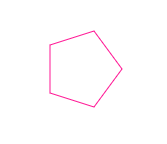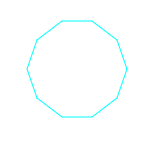CSC 240: Computer Graphics

Lab 2: Regular Polygon

in-class

This lab introduces regular polygons in a way that prepares you for transformations later on. It will also give you some experience with JavaScript "Math" functions and built-in line drawing. For this lab, use pair programming with your random partner (i.e. use one person's computer, then email the code to the other person afterward).

Step 1: Study the code

Download lab2.html (right-click on this link and then choose "Save Link As..."). Open this file in Sublime (or your preferred editor). Study the arguments to regularPolygon and make sure each of them makes sense. The first step is to set graphics.strokeStyle to the chosen line color.

Step 2: Compute θ

As discussed in class, we want to find θ such that θ*n = 360 degrees, where n is the number of sides of the polygon. We'll need to use radians in JavaScript, so you can use:

2*Math.PI // 360 degrees

Step 3: Compute the start point

When using lines to create a polygon in JavaScript, we need to specify the start point using graphics.moveTo(startX,startY). Uncomment this line and fill in the correct x and y values for the start point.

Step 4: For loop over the sides

Finally, create a for loop that goes over all n sides. Inside the for loop, first compute the angle based on θ*n. Then compute the x and y coordinates of the new point. Then use graphics.lineTo(x,y) to add this point to the path.

Call your regular polygon function from within draw(). Experiment with a variety of number of sides, sizes, and colors. Here are some examples with n=5 and n=10:You do not have to turn this lab in, but make sure both you and your pair programming partner have a copy by the end of the lab. We will build on this work for Homework 2 (filling the polygons!)